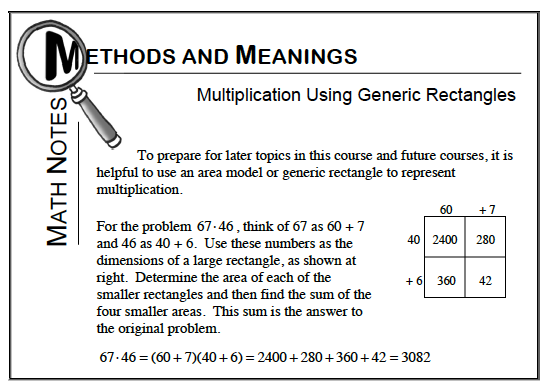### Home > CC1MN > Chapter 7 Unit 7 > Lesson CC1: 7.3.4 > Problem7-130

7-130.

Use a generic rectangle to multiply $(467)(392)$.

Refer to the Math Notes box below for how to sketch and use generic rectangles.
Be sure to check your work!$(467)(392) = 120,000 + 36,000 + 18,000 + 5400 + 2100 + 800 + 630 + 120 + 14 = 183,064$